# More on Functions Intro to Computer Science CS

• Slides: 23More on Functions Intro to Computer Science CS 1510 Dr. Sarah DiesburgFunctions From mathematics we know that functions perform some operation and return one value. They “encapsulate” the performance of some particular operation, so it can be used by others (for example, the len() function).Why Have Them? Abstraction of an operation Reuse: once written, use again Sharing: if tested, others can use Security: if well tested, then secure for reuse Simplify code: more readable Support divide-and-conquer strategy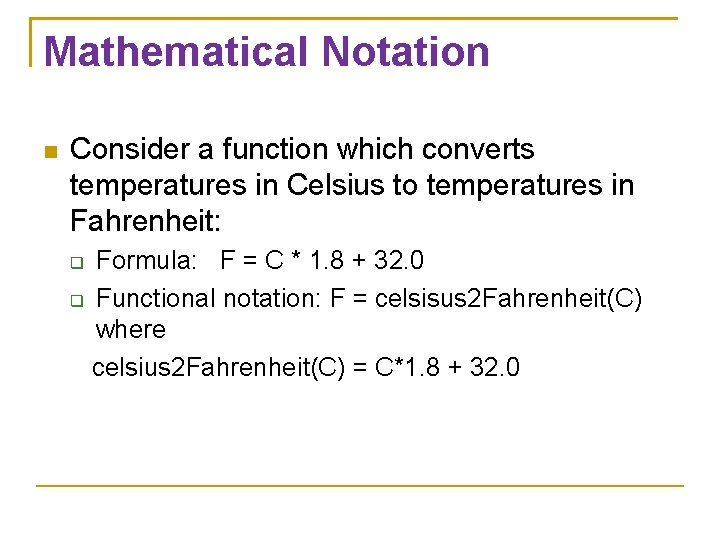Mathematical Notation Consider a function which converts temperatures in Celsius to temperatures in Fahrenheit: Formula: F = C * 1. 8 + 32. 0 Functional notation: F = celsisus 2 Fahrenheit(C) where celsius 2 Fahrenheit(C) = C*1. 8 + 32. 0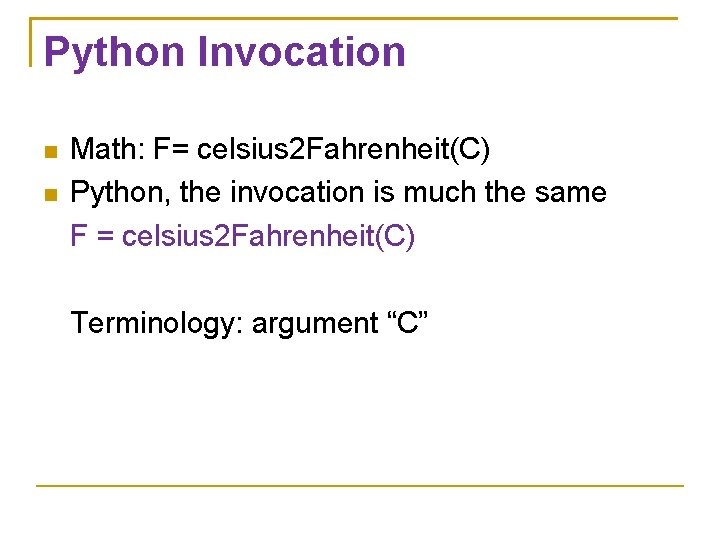Python Invocation Math: F= celsius 2 Fahrenheit(C) Python, the invocation is much the same F = celsius 2 Fahrenheit(C) Terminology: argument “C”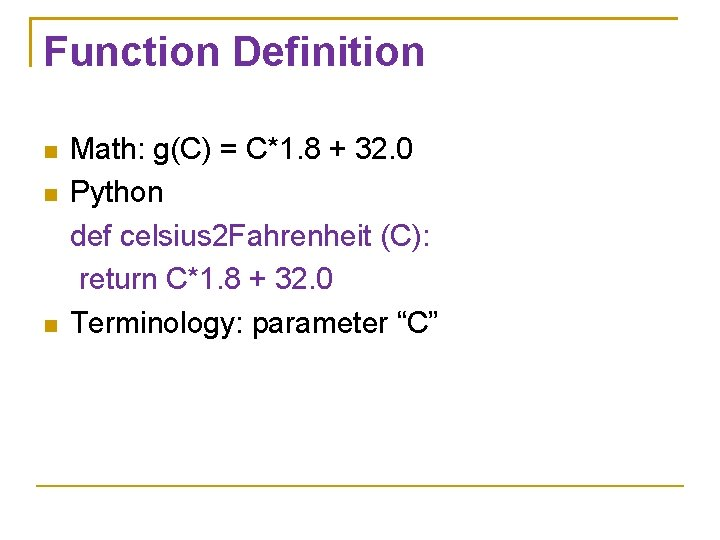Function Definition Math: g(C) = C*1. 8 + 32. 0 Python def celsius 2 Fahrenheit (C): return C*1. 8 + 32. 0 Terminology: parameter “C”Code Listing 6. 1 # Temperature conversion def celsius 2 fahrenheit(celsius): """ Convert Celsius to Fahrenheit. """ return celsius*1. 8 + 32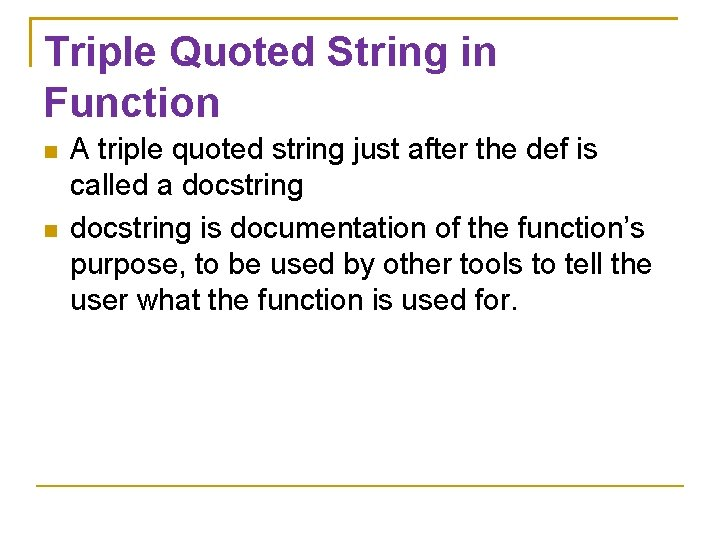Triple Quoted String in Function A triple quoted string just after the def is called a docstring is documentation of the function’s purpose, to be used by other tools to tell the user what the function is used for.Two Parts to a Function Definition – creates the function Invocation – is the application of a function within a program A function must be defined before it is invoked 9Function Definition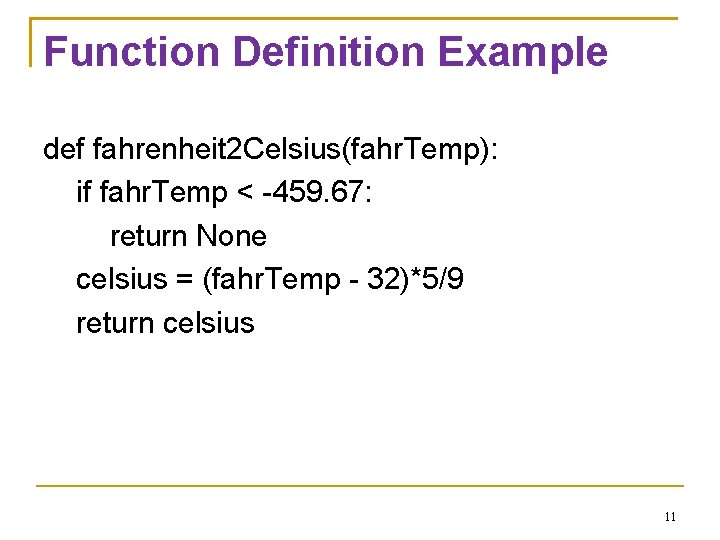Function Definition Example def fahrenheit 2 Celsius(fahr. Temp): if fahr. Temp < -459. 67: return None celsius = (fahr. Temp - 32)*5/9 return celsius 11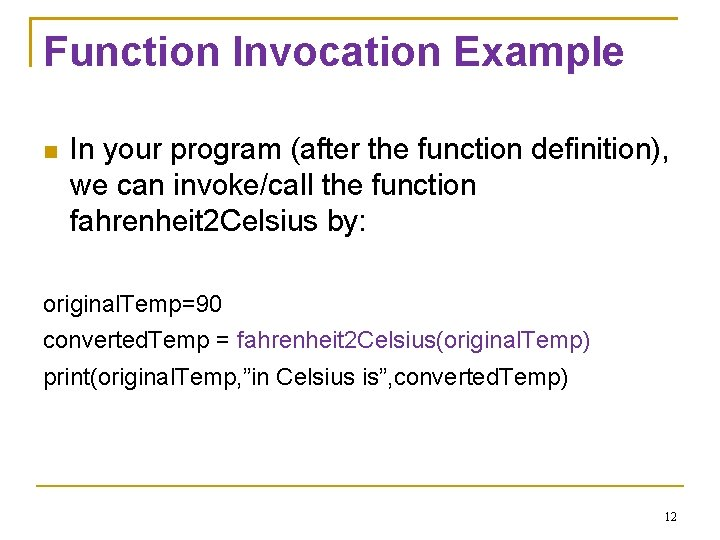Function Invocation Example In your program (after the function definition), we can invoke/call the function fahrenheit 2 Celsius by: original. Temp=90 converted. Temp = fahrenheit 2 Celsius(original. Temp) print(original. Temp, ”in Celsius is”, converted. Temp) 12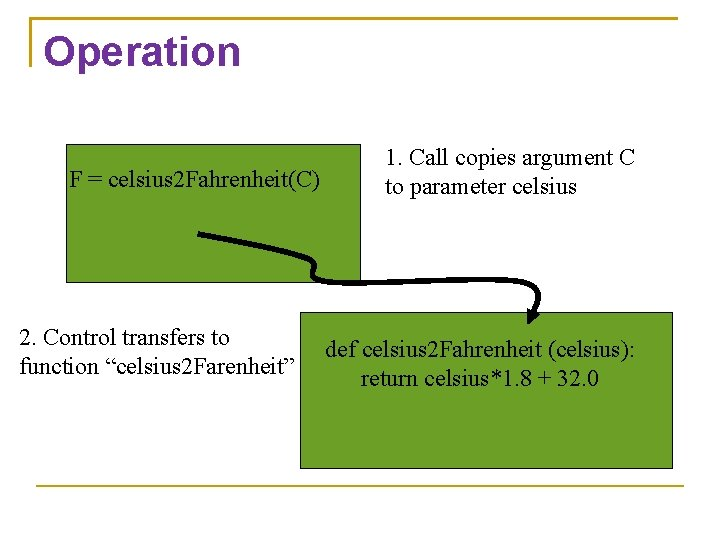Operation F = celsius 2 Fahrenheit(C) 2. Control transfers to function “celsius 2 Farenheit” 1. Call copies argument C to parameter celsius def celsius 2 Fahrenheit (celsius): return celsius*1. 8 + 32. 0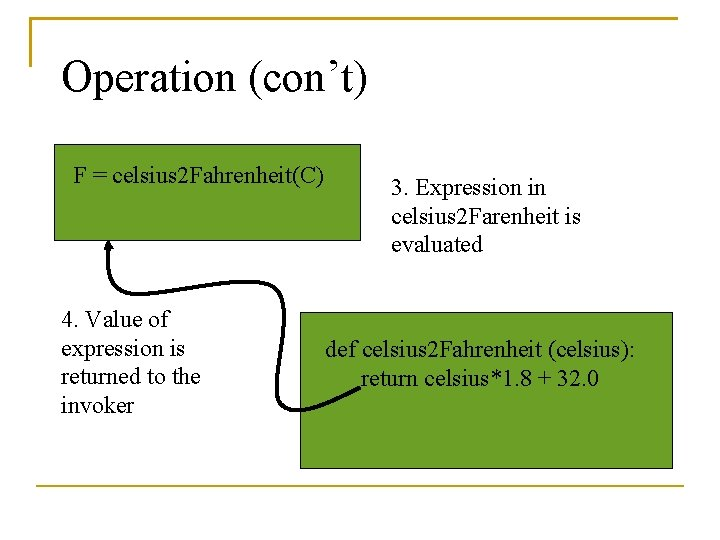Operation (con’t) F = celsius 2 Fahrenheit(C) 4. Value of expression is returned to the invoker 3. Expression in celsius 2 Farenheit is evaluated def celsius 2 Fahrenheit (celsius): return celsius*1. 8 + 32. 0Return Statement The return statement indicates the value that is returned by the function. The statement is optional (the function can return nothing). If no return, the function is often called a procedure.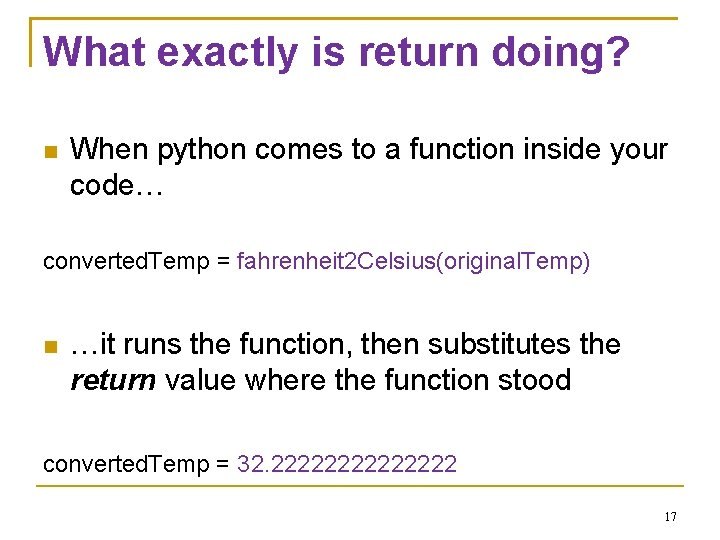What exactly is return doing? When python comes to a function inside your code… converted. Temp = fahrenheit 2 Celsius(original. Temp) …it runs the function, then substitutes the return value where the function stood converted. Temp = 32. 2222222 17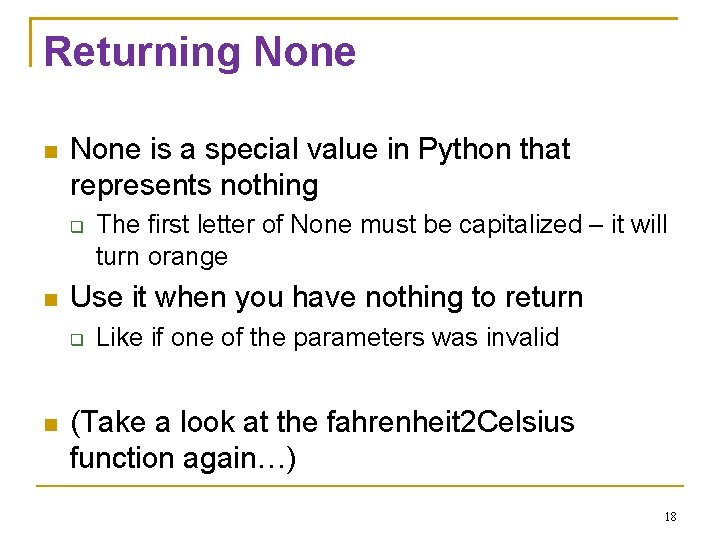Returning None is a special value in Python that represents nothing Use it when you have nothing to return The first letter of None must be capitalized – it will turn orange Like if one of the parameters was invalid (Take a look at the fahrenheit 2 Celsius function again…) 18Multiple Returns in a Function A function can have multiple return statements. Remember, the first return statement executed ends the function. #doing function stuff return result print(“Hello!”) #This line will never happenMultiple Returns in a Function When you use if/else statements, you could place a return in every branch. if result < 0: return None elif result == 1: return 1 else: return 42 #the answer to everything else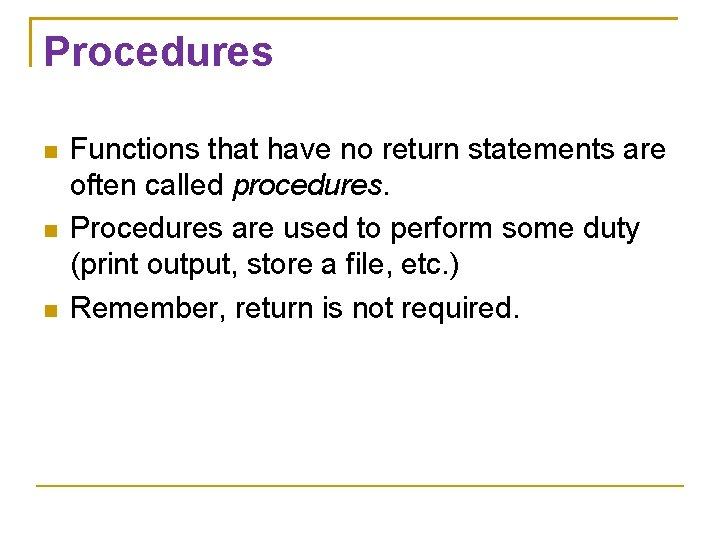Procedures Functions that have no return statements are often called procedures. Procedures are used to perform some duty (print output, store a file, etc. ) Remember, return is not required.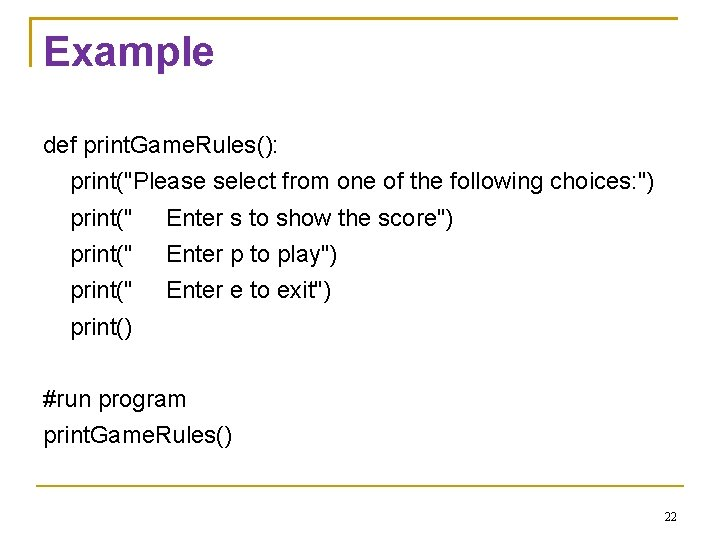Example def print. Game. Rules(): print("Please select from one of the following choices: ") print(" Enter s to show the score") print(" Enter p to play") print(" Enter e to exit") print() #run program print. Game. Rules() 22Functions can also call themselves One function can invoke another function Let’s take a look at function. Fun. py 23